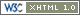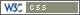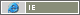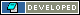Notes
Introduction
 Below is an article first published on the TML on 31 Jan 1995. Following the subsequent debate on the TML a revision was in order. That revision is appended below the main article. (Since "G" is used in the formula below, the unit of "G" is expressed in "gees" to avoid confusion.)
Original Article (aka "Simple Gravity" model)

Here's a thought or two ...

1) The jump drive's "100 diameter" safe jumping distance is supposed to be due to the gravity from large masses affecting the ability of the jump drive to form a jump field correctly. But gravity will vary with planetary density. According to "From Port to Jump-point" (JTAS 22) this number is a thumbnail rule anyway so what's the real rule?

2) In MT the jump task is 'Routine' outside this limit, 'Difficult' between 10 and 100 diameters, and 'Formidable' within 10 diameters. Each of these are a gap of 4 on the dice roll, yet the effect is described as gradual ("Starship Operator's Manual Vol 1") ... so does that mean its 'Difficult+2' at 50 diameters?

I propose that the phrase "100 diameter limit" is merely a rule of thumb provided by ship's pursers to their passengers and that seasoned space travellers have a more accurate value for the MSD (minimum safe distance) to form a jump point.

First some boring math so you can follow my reasoning if interested:

Using the formula

L = 64 (M / G)0.5

and

R = 8 D

from the original LBB rule books (where L is distance in mapping units, M is mass in earth masses, G is gravity in gees, R is world radius in mapping units, D is world UPP size code) you can deduce that 1 mapping unit = 62.5 miles (or approx 100 km as stated in the original book). So to express the formula for L in miles ...

L (in miles) = 4000 (M / G)0.5

or put another way

G = M / (L / 4000)2

Not wanting to be too terra-centric lets pick Vland as our reference example. (As an aside the specified mass for Vland given in ""Aliens Vol 1: Vilani & Vargr"" is incorrect - given a diameter of 14,850 km and a density of 1.02 the mass should be 1.5661792.)

So G at 100 diameters is 2.94185 x 10-5 gees. Remember from your high school physics that 1 gee is 9.80665 m/s/s and you get G = 2.88497 x 10-4 m/s/s.

Now because the the chance of a world being exact like that is small lets use some pseudo-science to pull a little slight-of-hand. The universally constant speed of light c = 2.99792458 x 108 m/s and so G/c is 9.62322 x 10-13 ... thats so close to 1 x 10-12 that maybe it should be! Now say that space stress is measured in units of S or in this case pS (p = 'pico' = 10-12) where 1 pS = 3.05703 x 10-5 gees.

So the 1 pS ('one pico ess') limit is the so-called '100 diameter' limit for Vland. Recalculating back gives 98.1 diameters.

So what was the point of all that (apart from wearing out my calculator)? Calculate the 1 pS boundary for Terra and you get 91.3 diameters ... not 100.

 Definition: Minimum safe distance to initiate Jump is any point in space where the gravitational stress factor is less than 1 pS.

 Definition: 1 pS is the stress on space by a gravitational force of 3.05703 x 10-5 gees.

Okay, so now we've dealt with the first point and can add some colour to individual systems.

 Sample Minimum Safe Distances World Icy Body Rocky Body Molten Core Heavy Core Size (k=0.32) (k=0.64) (k=0.96) (k=1.45) S 6.5 9.1 11.2 13.8 1 18.3 25.9 31.8 39.0 2 25.9 36.7 44.9 55.2 3 31.8 44.9 55.0 67.6 4 36.7 51.9 63.5 78.1 5 41.0 58.0 71.0 87.3 6 44.9 63.5 77.8 95.6 7 48.5 68.6 84.0 103.3 8 51.9 73.3 89.9 110.4 9 55.0 77.8 95.3 117.1 A 58.0 82.0 100.4 123.4
MSD in planetary diameters at 1 pS boundary
(incorrectly referred to as "100 diameter limit")

 Other Minimum Safe Distances Other Bodies Size MSD Small Gas Giant (k=0.20) 60 112.3 Large Gas Giant (k=0.20) 170 189.0 Star (Sol) (k=0.26) 865 482.6
Note that Sol's MSD of 482.6 is equavalent to 4.49 AU
(almost as far as Jupiter's orbit)!

Repeating this process for the "10 diameter" limit and we find its actually the 100 pS boundary. Hey, the numbers go up with the difficulty! A geometric progression gives a step of 3.1622777 or ...

 Jump Difficulty Stress Factorxxx MSD Difficulty < 1 pSxxxxx 100% Routine 1 pS - 3 pS 56% Routine+1 3 pS - 10 pS 32% Routine+2 10 pS - 32 pS 18% Routine+3 32 pS - 100 pS 10% Difficult 100 pS - 316 pS 6% Difficult+1 316 pS - 1,000 pS 3% Difficult+2 1,000 pS - 3,162 pS 2% Difficult+3 3,162 pS - 10,000 pS 1% Formidable 10,000 pS - 31,623 pS Formidable+1 31,623 pS - 100,000 pS Formidable+2 100,000 pS - 316,228 pS Formidable+3 316,228 pS
The MSD column assumes only one distorting object.

So if your players are running for the "100 diameter" MSD jump point while being persued but for feel the need to go early, just compare their distance with the table above to determine the actual risk at that point.

Another issue: Are Lagrange points (points of gravitational stability) safe jump points within the 1 pS boundary? No. In none of the published Traveller works is there any mention of this. The term ""stress"" is important, at these points the gravitational forces may be in balance but the fabric of space is still stressed (like the rope in a tug-o-war between two evenly matched teams). Therefore, even when two or more gravitational sources are balanced their effects are additive. Compute the pS value of all likely objects and add them together before consulting the Jump Difficulty Table.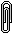ATTENTION In general, the Lagrange points don't have gravitational forces balanced. That can only happen at L1, and even there only if the 2 masses are equal. All other cases balance the 2 gravitational forces and the centrifugal force of the orbit.

 Range Stress MSD Stress Factor 5% 400.000 10% 100.000 15% 44.444 20% 25.000 25% 16.000 30% 11.111 35% 8.163 40% 6.250 45% 4.938 50% 4.000 55% 3.306 60% 2.778 65% 2.367 70% 2.041 75% 1.778 80% 1.563 85% 1.384 90% 1.235 95% 1.108 100% 1.000

Also note that this space stress does not simply end at 1 pS, but continues out indefinately getting smaller and smaller. It is theoretically possible to be beyond MSD for all objects yet their combined gravitational pulls to still exert more than 1 pS!

 Range Stress (cont) MSD Stress Factor 110% 0.826 120% 0.694 130% 0.592 140% 0.510 150% 0.444 160% 0.391 170% 0.346 180% 0.309 190% 0.277 200% 0.250

For example, at 140% MSD the stress factor is 0.510. So if a starship were at 140% MSD for one planet and 140% MSD for another planet, the total stress factor would be 1.100. This makes the jump task 'Routine +1'.

One final issue: When player characters are feeling particulaly suicidal/desperate/destructive they sometimes wonder what would happen if they tried to jump while still landed in the starport. On a 1 gee planet the stress factor at ground level is 32,711.463 pS which makes such a task 'Formidable +2'. Now you know!

Revision (aka "Tidal Force" model)

In the debate that followed this article being posted to the TML it was concluded that a simple gravity model for jump difficulty deviated from canon too much. The problem was that most Earth-like worlds were embedded very deeply within their star's MSD: calculating a mainworld's MSD was irrelevant. The reason is that simple gravity decreases with the square of the distance, making the MSD of large objects too big.

An alternative would be to use tidal force instead of simple gravity. Tidal force decreases with the cube of the distance and thus avoids this problem.

Boring math bit ...

For tidal force we'll use the formula:

F = N M / D3

And for no good reason we'll keep Vland's MSD at 98.1 diameters and the measure of stress at MSD as 1 pS (as in the original article). That means ...

D = 14850 x 98.1 = 1456785

1 pS = N x 1.5661792 / 14567853

therefore N = 1973990

F = 1973990 M / D3

or D = (1973990 M / F)(1/3)

(where D is in kilometers, M is in earths)

Since M is calculated from size, and we want D in terms of size, this factor drops out leaving us with ...

D = (925565 K / F)(1/3)

(where D is distance in diameters, K is density in earths, F is stress in pS)

Example: Vland K=1.02, F at MSD = 1 then ...

D = (925565 x 1.02 / 1)(1/3) = 98.1

 D = (925565 K / F)(1/3) F = 925565 K / D3 (where D is distance in diameters, K is density in earths, F is stress factor in pS)

So with the revised formula lets review the sample MSDs.

 Sample Minimum Safe Distances World Ice Body Rocky Body Molten Core Heavy Core Size (k=0.32) (k=0.64) (k=0.96) (k=1.45) any 66.7 84.0 96.1 110.3
MSD in planetary diameters at 1 pS boundary
(incorrectly referred to as "100 diameter limit")

 Other Minimum Safe Distances Other Bodies Size MSD Small Gas Giant (k=0.20) 60 57.0 Large Gas Giant (k=0.20) 170 57.0 Star (Sol) (k=0.26) 865 61.8

Sol's MSD by this method comes out as 61.8 diameters ... equivalent to 0.57 AU, or just past the orbit of Mercury. A big improvement on the original figure. Also, although there is now no variation based on world size there is still variation based on density ... and for a density of 1.08 the MSD really is 100 diameters!

Reanalysing for the "10 diameter" limit we find the stress is 1000 pS (not 100 pS). The stress factor is still increasing but step is now 5.623414. Recomputing the jump difficulties ...

 Jump Difficulty Stress Factorxxxxxxx MSD Difficulty < 1 pSxxxxxxxxx 100% Routine 1 pS - 6 pS 56% Routine+1 6 pS - 32 pS 32% Routine+2 32 pS - 178 pS 18% Routine+3 178 pS - 1,000 pS 10% Difficult 1,000 pS - 5,623 pS 6% Difficult+1 5,623 pS - 31,623 pS 3% Difficult+2 31,623 pS - 177,828 pS 2% Difficult+3 177,828 pS - 1,000,000 pS 1% Formidable 1,000,000 pS - 5,623,415 pS Formidable+1 5,623,415 pS - 31,622,791 pS Formidable+2 31,622,791 pS - 177,828,027 pS Formidable+3 177,828,027 pS
The MSD column assumes only one distorting object.

Finally, here is the recalculated Range Stress table:

 Range Stress MSD Stress Factor 5% 8000.001 10% 1000.000 15% 296.296 20% 125.000 25% 64.000 30% 37.037 35% 23.324 40% 15.625 45% 10.974 50% 8.000 55% 6.011 60% 4.630 65% 3.641 70% 2.951 75% 2.370 80% 1.953 85% 1.628 90% 1.372 95% 1.166 100% 1.000 110% 0.751 120% 0.579 130% 0.455 140% 0.364 150% 0.296 160% 0.244 170% 0.204 180% 0.171 190% 0.146 200% 0.125

Conclusion: Using a tidal force model rather than a simple gravity model gives us a set of figures which solve the original intent and conforms much more closely to canon. The only draw back is that without the G/c trick of the simple gravity model the MSD now seems to be arbitarily set. (But I guess we can't have everything.)